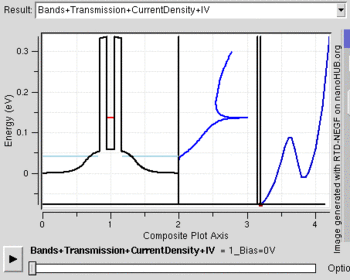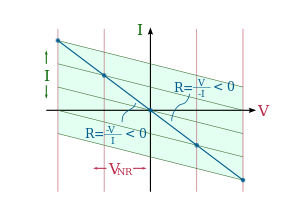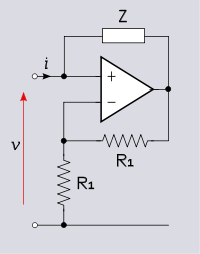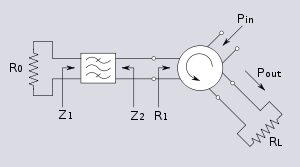# Negative resistance

Negative resistance

Negative resistance is a property of some electric circuits where an increase in the current entering a port results in a decreased voltage across the same port. This is in contrast to a simple ohmic resistor, which exhibits an increase in voltage under the same conditions. Negative resistors are theoretical and do not exist as a discrete component. However, some types of diodes (e.g., tunnel diodes) can be built that exhibit negative resistance in some part of their operating range. Such a differential negative resistance is illustrated in Figure 1 with a resonant-tunneling diode. Similarly, some chalcogenide glasses, organic semiconductors, and conductive polymers exhibit a similar region of negative resistance as a bulk property.Figure 1: A working mechanism of a Resonant Tunneling Diode device and negative differential resistance in output characteristic. Notice the negative resistance characteristic after the first current peak due to reduction of first energy level below source fermi level with gate bias.

## PropertiesFigure 2: The IV curve of a theoretical negative resistor

Fig. 2 shows a graph of a negative resistor, showing the negative slope. In contrast to this, a resistor will have a positive slope. Tunnel diodes and Gunn diodes exhibit a negative resistance region in their IV (current - voltage) curve. They have two terminals like a resistor; but are not linear devices. Unijunction transistors also have negative resistance properties when a circuit is built using other components.

For negative resistance to be present there must be active components in the circuit providing a source of energy. This is because current through a negative resistance implies a source of energy just as current through positive resistance implies that energy is being dissipated. A resistor produces voltage that is proportional to the current through it according to Ohm's law. The IV curve of a true negative resistor has a negative slope and passes through the origin of the coordinate system (the curve can only enter the 2nd and 4th quadrants if energy is being supplied). This is to be compared with devices such as the tunnel diode where the negative slope portion of the curve does not pass through the origin. Clearly, there is no source of energy in a two terminal diode.

## History

In early research it was noticed that arc discharge devices and some vacuum tube devices such as the dynatron exhibit negative differential resistance effects. Practical and economic devices only became available with solid state technology. The typical true negative impedance circuit—the negative impedance converter -- is due to John G. Linvill (1953) and the popular element with negative differential resistance—the tunnel diode -- is due to Leo Esaki (1958).

## ImplementationsFigure 3: Negative impedance circuit with$Z_{\text{in}} \triangleq \frac{v}{i} = -Z$

### Diodes

Tunnel diodes are heavily doped semiconductor junctions that have an "N" shaped transfer curve. A vacuum tube can also be made to exhibit negative resistance. Other negative resistance diodes have been built that have an "S" shaped transfer curve. When biased so that the operating point is in the negative resistance region, these devices can be used as an Amplifier. These devices can also be biased so that they will switch between two states very quickly, as the applied voltage changes.

### Operational Amplifiers

The negative resistance circuit shown in Figure 3 is an opamp implementation of the negative impedance converter (see below). The two resistors R1 and the op amp constitute a negative feedback non-inverting amplifier with gain A = 2. In the case Z = R, the input resistance (for an ideal opamp) is given by;$R_\text{in} = -Z = -R \,\!$

The input port of the circuit can be connected into another network as if it were a negative resistance component.

In the general case Z can be selected to produce negative capacitances or negative inductances.

## Applications

### Oscillators

All feedback oscillators imply the presence of negative resistance. There are many such topologies, including the Dynatron oscillator, Colpitts oscillator, Hartley oscillator, Wien bridge oscillator, and some types of relaxation oscillators. If the feedback loop is broken and the input impedance examined it will be found to include negative resistance. Negative resistance characteristics of Gunn diodes are often used in microwave frequencies as well.

### AmplifiersFigure 4: Negative resistance microwave amplifier using a circulator
File:10Gig Tunnel Amp S.jpg
Figure 5: 8 - 12 GHz tunnel diode amplifier, circa 1970

A device exhibiting negative resistance can be used to amplify a signal and this is an especially useful technique at microwave frequencies. Such devices do not present as pure negative resistance at these frequencies (in the case of the tunnel diode a large parallel capacitance is also present) and a matching filter is usually required. The reactive components of the device's equivalent circuit can be absorbed into the filter design so the circuit can be represented as a pure resistance followed by a bandpass filter. The output of this arrangement is fed into one port of a three-port circulator. The other two ports constitute the input and output of the amplifier with the direction of circulation as shown in the diagram. Treating R0 as being positive, the reflection coefficients at the two ends of the filter are given by;$\Gamma_1 = \frac{Z_1 - R_0}{Z_1 + R_0}$ and,$\Gamma_2 = \frac{Z_2 - R_1}{Z_2 + R_1}$

Since the filter has no resistive elements, there is no dissipation and the magnitudes of the two reflection coefficients must be equal,$\left| \Gamma_1 \right| = \left| \Gamma_2 \right|$

The input power entering the cirulator is directed at the matching filter, is reflected at both the input and output of the filter and a portion finally arrives at the load. This portion is given by;$\frac{P_\mbox{out}}{P_\mbox{in}} = \left| \Gamma_1 \right|^2$

For a well matched filter, the reflection coefficients will be very small in the passband and very little power will reach the load. On the other hand if R0 is replaced by a negative resistance such that,$R_0' = - R_0 \,\!$ then,$\Gamma_1' = \frac{Z_1 + R_0}{Z_1 - R_0}$ and,$\left| \Gamma_1' \right| = \left| \Gamma_2' \right| = \frac{1}{\left| \Gamma_1 \right|}$

Now the reflection coefficients are very large and more power is reaching the load than was injected in the input port. The net result of terminating one port in a negative resistance is amplification between the remaining two ports.

### Mixers and frequency converters

The highly non-linear characteristics of tunnel diodes makes them useful as frequency mixers. The conversion gain of a tunnel diode mixer can be as high as 20 dB if it is biased to operate in the negative resistance region.

### Antenna design

Another concept of negative resistance exists in the domain of radio frequency antenna design. This is also known as negative impedance. It is not uncommon for an antenna containing multiple driven elements to exhibit apparent negative impedance in one or more of the driven elements.

### Impedance cancellation

Negative impedances can be used to cancel the effects of positive impedances, for example, by eliminating the internal resistance of a voltage source or making the internal resistance of a current source infinite. This property is used in telephony line repeaters and in circuits such as the Howland current source, Deboo integrator and load cancellers.

Wikimedia Foundation. 2010.

### Look at other dictionaries:

• negative resistance — neigiamoji varža statusas T sritis fizika atitikmenys: angl. negative resistance vok. negativer Widerstand, m; Negwid, m rus. отрицательное сопротивление, n pranc. résistance négative, f …   Fizikos terminų žodynas

• negative resistance — noun : a resistance phenomenon (as exhibited by an electric arc or vacuum tube) in which the voltage drop across the circuit decreases as the current increases …   Useful english dictionary

• negative resistance — /nɛgətɪv rəˈzɪstəns/ (say neguhtiv ruh zistuhns) noun the property of certain devices in which an increase in the voltage applied causes a decrease in the current passing through them …   Australian-English dictionary

• negative resistance effect — neigiamosios varžos reiškinys statusas T sritis automatika atitikmenys: angl. negative resistance effect vok. negativer Widerstandseffekt, m rus. эффект отрицательного сопротивления, m pranc. effet de résistance négative, m …   Automatikos terminų žodynas

• negative-resistance device — neigiamosios varžos įtaisas statusas T sritis radioelektronika atitikmenys: angl. negative resistance device vok. Bauelement mit negativem Widerstand, n rus. прибор с отрицательным сопротивлением, m pranc. dispositif à résistance négative, m …   Radioelektronikos terminų žodynas

• negative-resistance diode — neigiamosios varžos diodas statusas T sritis radioelektronika atitikmenys: angl. negative resistance diode vok. Diode mit negativem Widerstand, f; Negativwiderstandsdiode, f rus. диод с отрицательным сопротивлением, m pranc. diode à résistance… …   Radioelektronikos terminų žodynas

• negative-resistance element — neigiamosios varžos elementas statusas T sritis radioelektronika atitikmenys: angl. negative resistance element vok. Element mit negativem Widerstand, n rus. элемент с отрицательным сопротивлением, m pranc. élément à résistance négative, m …   Radioelektronikos terminų žodynas

• negative-resistance characteristic — neigiamosios varžos charakteristika statusas T sritis radioelektronika atitikmenys: angl. negative resistance characteristic vok. fallende Widerstandscharakteristik, f rus. характеристика с отрицательным сопротивлением, f pranc. caractéristique à …   Radioelektronikos terminų žodynas

• negative resistance region — neigiamosios varžos sritis statusas T sritis fizika atitikmenys: angl. negative resistance region vok. Bereich des negativen Widerstandes, m rus. область отрицательного сопротивления, f pranc. région de résistance négative, f …   Fizikos terminų žodynas

• negative resistance training — neigiamojo pasipriešinimo pratybos statusas T sritis Kūno kultūra ir sportas apibrėžtis Jėgos ugdymo pratybos, kuriose vyrauja ekscentrinė raumenų veikla, pvz., aukštai pakeltos štangos nuleidimas, bėgimas į pakalnę. atitikmenys: angl. negative… …   Sporto terminų žodynas